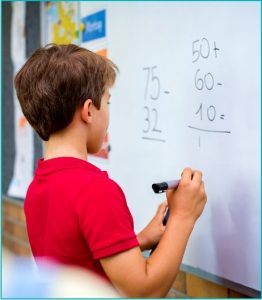# Math Level A (formerly Level 1)

In response to customer feedback, we have expanded and re-levelled our math program for Fall-Winter 2023-24. As a result, some of our courses have been renamed for 2023-24.Our Level A Math & Problem-Solving Program (formerly Level 1) typically targets students in Grades 4 through 6. (Admission into Level A is not restricted to the above grade levels but is based on student ability and readiness).

The major emphasis at this level of program is learning how to think critically in understanding the problem at hand. Attention is given to recognizing different problem-solving strategies and applying logic strategies to solve these to problems. We aim to give students the tools to solve problems using their mathematical knowledge. Students will learn to think abstractly, collaborate with others, persevere, and approach problems from different perspectives. Lessons include interactive puzzles, brain teasers, logic problems, and competitions to help students stay sharp, discover new strategies, and develop mathematical thinking through numerous approaches.

Level A instruction includes, but is not limited to:

Math

• Understanding numerical patterns
• Divisibility and factors
• Four operations (+,-, x, /,) on multi digit whole numbers
• Four operations on decimals
• Four operations on fractions
• Percent equivalence and percent of a number
• Order of operations involving whole numbers, decimals, fractions, and percent
• Perimeter and area

Strategy games

• Magic squares
• Crack the code
• Find the mystery number
• Sudoku
• Mazes
• Optical illusions
• Card games
• Competitions
• Hands-on fun

Memory development

• Brain Power memory technique

Logic and Problem-Solving Strategies

• Introduction of the 4-step problem solving practice
• Modeling problems and simplifying them
• Guess and check
• Forward solving strategies
• Backwards solving strategies
• Venn diagrams
• Logical trees
• Logical charts
• Organized / systematic list
• Problems on lowest common multiple
• Discovering patterns

Prerequisite for Level 1: knowledge of the multiplication table through 10 and good reading skills.